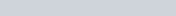ChemTalk

# Dalton’s Law of Partial Pressure

## Dalton’s Law Core Concepts

In 1801, English chemist John Dalton made observations about steam and air, that is published in 1802 and eventually because Dalton’s law of partial pressure. In this tutorial, you will learn what partial pressure is, how to find the partial pressure of a gas using the partial pressure formula, and how Dalton’s Law relates it to mole fraction.

## Multiple Gases

Consider a tank containing 1 mole of neon gas. Let the pressure be 1-atmosphere. Now, consider what would happen if you added another mole of neon gas.

From our article on pressure, What is Pressure, we know that pressure is caused by the particles of gas colliding against the container walls. Because doubling the amount of moles of gas would double the amount of gas particles, we know that adding this second mole of gas would double the pressure.

Now, consider what would happen if instead of adding another mole of neon gas, we added a mole of helium. The amount of particles in a mole is the same no matter the substance, so we know that the amount of particles we’re adding is the same as before.

Thus, we can conclude that by adding more of a different gas, the pressure of the tank will increase.

## Partial Pressure

In the tank from before containing neon and helium, the pressure would be 2-atmospheres, because the amount total amount of moles is 2 moles, and each mole exerts one atmosphere.

From this conclusion, we can see that the amount of pressure exerted by each species of gas is not the full 2 atmospheres, but is instead a portion of the entire pressure.

## Dalton’s Law of Partial Pressure

Dalton’s Law of Partial Pressure states that the sum of these portions add up to the entire pressure of the container — i.e., the sum of the pressures of the neon and helium from the tank before add up to the total pressure of the tank.

From this, we can see the equation arise: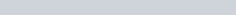. This equation is Dalton’s Law, and it can be used to determine the total pressure of a system.

## The Partial Pressure Formula

The partial pressure formula is a way to calculate the pressure that a gas would have if it were the only gas present in a mixture of gases. This is useful because the total pressure of a gas mixture is the sum of the partial pressures of all the gases in the mixture.

To use the formula, you need to know the fraction of a gas in the mixture and the total pressure of the gas mixture. The fraction of a gas in the mixture is the ratio of the number of molecules of that gas to the total number of molecules in the mixture. For example, if a gas mixture contains 25 molecules of one gas and 75 molecules of another gas, the fraction of the first gas in the mixture would be 0.25.

Once you have the fraction of a gas in the mixture and the total pressure of the gas mixture, you can use the partial pressure formula to calculate the partial pressure of that gas. The formula is as follows:

Partial pressure = (fraction of gas in the mixture) * (total pressure of the gas mixture)

For example, if the fraction of a gas in a mixture is 0.25 and the total pressure of the gas mixture is 100 kPa, then the partial pressure of that gas would be 25 kPa.

## Mole Fractions and Partial Pressure

Before, we were adding in equal portions of gas. What would happen if we added unequal portions instead?

Consider the same tank from before, except instead of 1 mole of neon gas, we add 3 moles originally. From Avogadro’s Law, we know that the pressure will be proportional to the amount of moles, so in this case the pressure of the neon gas will be 3 moles.

Next, we add 1 mole of helium gas. Now, the total amount of moles of gas isn’t doubled, but is instead increased by 33%. Because of this, there are only 33% more collisions with the container walls, and thus the pressure only increases by 1 atm.

From this, we can see that the overall pressure becomes 4 atm, and the total moles becomes 4 moles. We can take the mole fraction of the helium gas,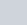, and see that it is equal to 0.25. This also is the fraction of pressure that is exerted by the helium gas.

Because of this, the partial pressure of a gas can be determined by its mole fraction through the equation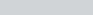, where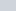is the mole fraction of gas 1.

## Dalton’s Law Example Problem

### How to find the Partial Pressure

Here’s an example of how you can solve a problem about Dalton’s Law of Partial Pressure, and how to find the partial pressure of a gas.

A tank is filled with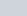of oxygen gas, and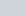of helium gas. The total pressure in the tank is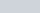. What are the partial pressures of the oxygen and helium gas?

First, determine the amount of moles of both the oxygen and helium gas.Then, determine the mole fractions of both the oxygen and helium gas.Finally, use the mole fraction to determine the partial pressure.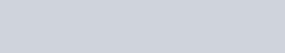## Dalton’s Law Practice Problems

Problem 1

You have a container with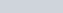of gaseous ethanol and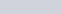of O2. You then combust as much ethanol as possible into carbon dioxide and water vapor. What is the total change in pressure (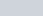) inside of the container after combustion? (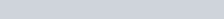}

Ethanol combusts according to the following equation: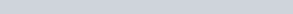Problem 2

You have a container with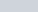each of N2, O2, and Ar with a total pressure of. You decide to add Ar to increase the total pressure to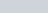. What is the final mole fraction and final partial pressure of Ar?

## Dalton’s Law Practice Problem Solutions

1: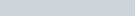2: